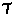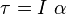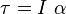Rotational Inertia, Torque and Angular Acceleration (1Q)

Homework Statement

. What is the angular acceleration if a force of 3 N is applied perpendicular to the radius of a 5 kg solid sphere that has a radius of 0.6 meters?

Homework Equations= r x F
I = mr^2

The Attempt at a Solution

I usedtried solving for angular acce.

Also, if the question had said "hollow sphere" would the moment of inertia be different..(2/3)mr^2 ?

TSny
Homework Helper
Gold Member
•Sam Cepeda
SteamKing
Staff Emeritus
Homework Helper

Homework Statement

. What is the angular acceleration if a force of 3 N is applied perpendicular to the radius of a 5 kg solid sphere that has a radius of 0.6 meters?

Homework Equations= r x F
I = mr^2

The Attempt at a Solution

I usedtried solving for angular acce.
•